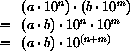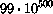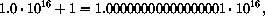# Section 33Intermezzo 6: The Nature of Inexact Numbers

Computers represent and process information in chunks of a fixed size. Because computers were first used for numerical calculations, early computer engineers developed a representation for numbers in terms of fixed-size chunks. Programming languages must mediate the gap between these fixed-size representations and the true mathematics. Because using the hardware representation for numbers makes a program's calculations as efficient as possible, most designers and implementors of programming languages adopted the hardware-based choice.

This intermezzo explains the fixed-size representation for numbers and its consequences in some detail. The first subsection introduces a concrete fixed-size representation for numbers, discusses what it implies for the representation of numbers, and shows how to calculate with such numbers. The second and third section illustrate the two most fundamental problems of fixed-size number arithmetic: overflow and underflow, respectively.

## 33.1  Fixed-size Number Arithmetic

Suppose we can use four digits to represent numbers. If we represent natural numbers, the representable range is 0 ...9999. Alternatively we could represent 10,000 fractions between 0 and 1 with that many digits. In either case, this is a rather small range of numbers and not useful for most scientific or business computations.

We can represent a larger range of numbers if we use a different notation for numbers instead. In science, for example, we encounter so-called scientific notation, which represents numbers as two parts:

1. a MANTISSA, which is a base number, and

2. an EXPONENT, which is used to determine a 10-based factor.

For pure scientific notation, the base is between 0 and 9. We relax this constraint and just write numbers aswhere m is the mantissa and e the exponent. For example, one representation of 1200 with this scheme isanother one isIn general, a number has several equivalents in mantissa-exponent representation.

We can also use negative exponents, which add fractions at the cost of one extra piece of data: the sign of the exponent. For example,stands forAs before, the fraction has several representations in the new notation.

To use a form of mantissa-exponent notation for our problem, we must decide how many digits we wish to use for the representation of the mantissa and how many for the exponent. Here we use two for each and a sign for the exponent; other choices are possible. Given this decision, we can still represent 0 asThe maximal number we can represent iswhich is 99 followed by 99 0's. If we use negative exponents in addition to positive ones, we can also representwhich is a small number close to 0. Thus we can now represent a vastly larger range of numbers with four digits and a sign than before. But this improvement comes with its own problems.

 ```;; `create-inex : N N N  ->  inex` ;; to make an instance of `inex` after checking the appropriateness ;; of the arguments (define (create-inex m s e) (cond [(and (<= 0 m 99) (<= 0 e 99) (or (= s +1) (= s -1))) (make-inex m s e)] [else (error 'make-inex "(<= 0 m 99), +1 or -1, (<= 0 e 99) expected")])) ;; `inex->number : inex  ->  number` ;; to convert an `inex` into its numeric equivalent (define (inex->number an-inex) (* (inex-mantissa an-inex) (expt 10 (* (inex-sign an-inex) (inex-exponent an-inex))))) ``` Figure 94:  Functions for inexact representations

To understand the problems, it is best to agree on a fixed representation schema and to experiment with the number representations. Let's represent a fixed-size number with a structure that has three fields:

```(define-struct inex (mantissa sign exponent))
```

The first and last field contain the mantissa and exponent of the number, the `sign` field is `+1` or `-1` and represents the sign of the exponent. This sign field enables us to represent numbers between `0` and `1`.

Here is the data definition:

An `inex` is a structure:

`(make-inex m s e)`
where `m` and `e` are natural numbers in [`0`,`99`] and `s` is `+1` or `-1`.

Because the conditions on the fields of an `inex` structure are so stringent, we use the function `create-inex` to create these structures. Figure 94 contains the function definition for `create-inex`, which is a generalized constructor, that is, a checked constructor (see section 7.5). The figure also defines the function `inex->number`, which turns `inex`s into numbers according to the principles of our new notation.

Let's translate the above example, `1200`, into our Scheme representation:

```(create-inex 12 +1 2)
```

The alternative representation, 120 · 101, is illegal in our Scheme world, however. If we evaluate

```(create-inex 120 +1 1)
```

we get an error message because the arguments don't satisfy the stated data contract. For other numbers, though, we can find two `inex` equivalents. One example is `0.0000000000000000005`, which we can express as

```(create-inex 50 -1 20)
and
(create-inex 5 -1 19)
```

Confirm the equivalence of these two representations with `inex->number`.

The range of `inex` numbers is vast:

```(define MAX-POSITIVE (make-inex 99 +1 99))
(define MIN-POSITIVE (make-inex 1 -1 99))
```

That is, we can represent large numbers that consist of up to 101 digits in the standard decimal notation; we can also represent small positive fractions smaller than `1` down to the fraction 1 over 10...0 with 99 zeros. The appearances, however, are deceiving. Not all real numbers in the range between 0 and `MAX-POSITIVE` can be translated into an `inex` structure. In particular, any positive number less thanhas no equivalent `inex` structure. Similarly, the `inex` representation has gaps in the middle. For example, the successor of

```(create-inex 12 +1 2)
```

is

```(create-inex 13 +1 2)
```

The first `inex` structure corresponds to `1200`, the second one to `1300`. Numbers in the middle, such as `1240` or `1260`, can only be represented as one or the other. The standard choice is to round the number to the closest representable equivalent. In short, we must approximate such mathematical numbers as we translate into a chosen representation.

Finally, we must also consider arithmetic operations on `inex` structures. Adding two `inex` representations with the same exponent means adding the two mantissas:

```  (inex+ (create-inex 1 +1 0)
(create-inex 2 +1 0))
= (create-inex 3 +1 0)
```

Translated into mathematical notation, we haveWhen the addition of two mantissas yields too many digits, we may have to find a suitable representation. Consider the example of addingto itself. Mathematically we getbut we can't just translate this number naively into our chosen representation because 110 > 99. The proper corrective action is to represent the result asOr, translated into Scheme, we must ensure that `inex+` computes as follows:

```  (inex+ (create-inex 55 +1 0)
(create-inex 55 +1 0))
= (create-inex 11 +1 1)
```

More generally, if the mantissa of the result is too large, we must divide it by `10` and increase the exponent by one.

Sometimes the result contains more mantissa digits than we can represent. In those cases, `inex+` must round to the closest equivalent in the `inex` world. For example:

```  (inex+ (create-inex 56 +1 0)
(create-inex 56 +1 0))
= (create-inex 11 +1 1)
```

This corresponds to the precise calculation:Because the result has too many mantissa digits, the integer division of the result mantissa by `10` produces an approximate result:This is an example of the many approximations that make INEXACT ARITHMETIC inexact.

We can also multiply numbers represented as `inex` structures. Recall thatThus we get:or, in Scheme notation:

```  (inex* (create-inex 2 +1 4)
(create-inex 8 +1 10))
= (make-inex 16 +1 14)
```

As with addition, things are not always straightforward. When the result has too many significant digits in the mantissa, `inex*` has to increase the exponent:

```  (inex* (create-inex 20 -1 1)
(create-inex  5 +1 4))
= (create-inex 10 +1 4)
```

In the process, `inex*` will introduce an approximation if the true mantissa doesn't have an exact equivalent in the class of `inex` structures:

```  (inex* (create-inex 27 -1 1)
(create-inex  7 +1 4))
= (create-inex 19 +1 4)
```

Exercise 33.1.1.   Develop the function `inex+`, which adds `inex` representations that have the same exponent. The function must be able to deal with examples that increase the exponent. Furthermore, it must signal its own error if the result is out of range for `inex` representations.

Challenge: Extend `inex+` so that it can deal with inputs whose exponents differ by `1`:

```(equal? (inex+ (create-inex 1 +1 0) (create-inex 1 -1 1))
(create-inex 11 -1 1))
```

Do not attempt to deal with larger classes of inputs than that without reading the following subsection.Solution

Exercise 33.1.2.   Develop the function `inex*`, which multiplies `inex` representations. The function must be able to deal with examples that increase the exponent. Furthermore, it must signal its own error if the result is out of range for `inex` representations.Solution

Exercise 33.1.3.   The section illustrated how an inexact representation system for real numbers has gaps. For example, 1240 was represented as ```(create-inex 12 +1 2)``` by rounding off the last significant digit of the mantissa. The problem is, round-off errors can accumulate.

Develop the function `add`, which adds up `n` copies of `#i1/185`. What is the result for `(add 185)`? What should it be? What happens if we multiply the result of the second expression with a large number?

Develop the function `sub`, which counts how often `1/185` can be subtracted from the argument until the argument is `0`. How often should the evaluation recur before `(sub 1)` and ```(sub #i1.)``` is evaluated? What happens in the second case? Why?Solution

## 33.2  Overflow

While the use of scientific notation expands the range of numbers we can represent with fixed-size chunks of data, it still doesn't cover arbitrarily large numbers. Some numbers are just too big to fit into a fixed-size number representation. For example,can't be represented, because the exponent 500 won't fit into two digits, and the mantissa is as large as it can be.

Numbers that are too large for our representation schema can arise during a computation. For example, two numbers that we can represent can add up to a number that we cannot represent:

```  (inex+ (create-inex 50 +1 99)
(create-inex 50 +1 99))
= (create-inex 100 +1 99)
```

which violates the data contract, or

```  (inex+ (create-inex 50 +1 99)
(create-inex 50 +1 99))
= (create-inex 10 +1 100)
```

which also breaks the contract for `inex` structures. When the result of `inex` arithmetic produces numbers that are too large to be represented, we say (arithmetic) OVERFLOW occurred.

When overflow occurs, some language implementations signal an error and stop the computation. Others designate some symbol, called infinity, for all numbers that are too large. Arithmetic operations are aware of infinity and propagate it.

Negative Numbers: If our `inex` structures had a sign field for the mantissa, then two negative numbers can add up to one that is so negative that it can't be represented either. This is also called overflow, though to emphasize the distinction people sometimes say overflow in the negative direction.

Exercise 33.2.1.   DrScheme's inexact number system uses an infinity value to deal with overflow. Determine the integer `n` such that ```(expt #i10. n)``` is still an inexact Scheme number and ```(expt #i10. (+ n 1))``` is approximated with infinity. Hint: Use a function to compute `n`.Solution

## 33.3  Underflow

At the opposite end of the spectrum, we have already seen small numbers that cannot be represented with `inex` structures. For example, 10-500 is not 0, but it's smaller than the smallest non-zero number we can represent. An arithemtic UNDERFLOW arises when we multiply two small numbers and the result is too small to fit into our class of `inex` structures:

```  (inex* (create-inex 1 -1 10)
(create-inex 1 -1 99))
= (create-inex 1 -1 109)
```

which causes an error.

When underflow occurs, some language implementations signal an error; others use 0 to approximate the result. An approximation with 0 for underflow is qualitatively different from our ealier kinds of approximations. In approximating 1250 with `(create-inex 12 +1 2)`, we approximated by dropping significant digits from the mantissa, but we were left with a non-zero mantissa. The result is within 10% of the number we wanted to represent. Appromixating on underflow, however, means dropping the entire mantissa. The result is not within a predictable precentage range of the true result.

Exercise 33.3.1.   DrScheme's inexact number system uses `#i0` to approximate underflow. Determine the smallest integer `n` such that `(expt #i10. n)` is still an inexact Scheme number and `(expt #i10. (- n 1))` is approximated with `0`. Hint: Use a function to compute `n`.Solution

## 33.4  DrScheme's Numbers

Most programming languages support only inexact number representations (and arithmetic) for both integers and reals. Scheme, in contrast, supports both exact and inexact numbers and arithmetic. Of course, the base of the representation is 2, not 10, because Scheme uses the underlying computer's on-off machinery.

As the note on page 5 explained, DrScheme's teaching levels interpret all numbers in our programs as exact rationals, unless they are prefixed with `#i`. Some numeric operations, though, produce inexact numbers. Plain Scheme, which is called Full Scheme in DrScheme, interprets all numbers with a dot as inexact numbers;66 it also prints inexact reals with just a dot, implying that all such numbers are inexact and possibly distant from the actual result.

Scheme programmers can thus choose to use exact arithmetic or inexact arithmetic as necessary. For example, numbers in financial statements should always be interpreted as exact numbers; arithmetical operations on such numbers should be as precise as possible. For some problems, however, we may not wish to spend the extra time to produce exact results. Scientific computations are a primary example. In such cases, we may wish switch to inexact numbers and arithmetic.

Numerical Analysis: When we use inexact numbers and arithmetic, it is natural to ask how much the program's results differs from the true results. Over the past few decades, the study of this complex question has evolved into an advanced topic, called numerical analysis. The discipline has become a subject of its own right in applied mathematics or in computer science departments.

Exercise 33.4.1.   Evaluate

```(expt 1.001 1e-12)
```

in Full Scheme (any variant) and in Intermediate Student Scheme. Explain the observations.Solution

Exercise 33.4.2.   Develop the function `my-expt`, which raises one number to the power of some integer. Using this function, conduct the following experiment. Add

```(define inex (+ 1 #i1e-12))
(define exac (+ 1 1e-12))
```

to the Definitions window. What is `(my-expt inex 30)`? How about `(my-expt exac 30)`? Which answer is more useful?Solution

Exercise 33.4.3.   When we add two inexact numbers of vastly different orders of magnitude, we may get the larger one back as the result. For example, if we are using only 15 significant digits, then we run into problems when adding numbers which vary by more than a factor of 1016:but if the number system supports only 15 digits, the closest answer is 1016. At first glance, this doesn't look too bad. After all, being wrong by one part in 1016 (ten million billion) is close enough to the accurate result. Unfortunately, this kind of problem can add up to huge problems.

Consider the following list of inexact numbers:

```(define JANUS
(list #i31
#i2e+34
#i-1.2345678901235e+80
#i2749
#i-2939234
#i-2e+33
#i3.2e+270
#i17
#i-2.4e+270
#i4.2344294738446e+170
#i1
#i-8e+269
#i0
#i99))
```

Determine the values `(sum JANUS)` and `(sum (reverse JANUS))`. Explain the difference. Can we trust computers?Solution

66 We can force Full Scheme to interpret numbers with a dot as exact by prefixing the numbers with #e.